# Price Indices

A measure of how prices change over a period of time

## What are Price Indices?

A price index (PI) is a measure of how prices change over a period of time, or in other words it is a way to measure inflation. There are multiple methods on how to calculate the inflation (or deflation), in this guide we will take a look at a couple of methods on how to do so. Inflation is one of the core metrics monitored by the FED in order to set interest rates.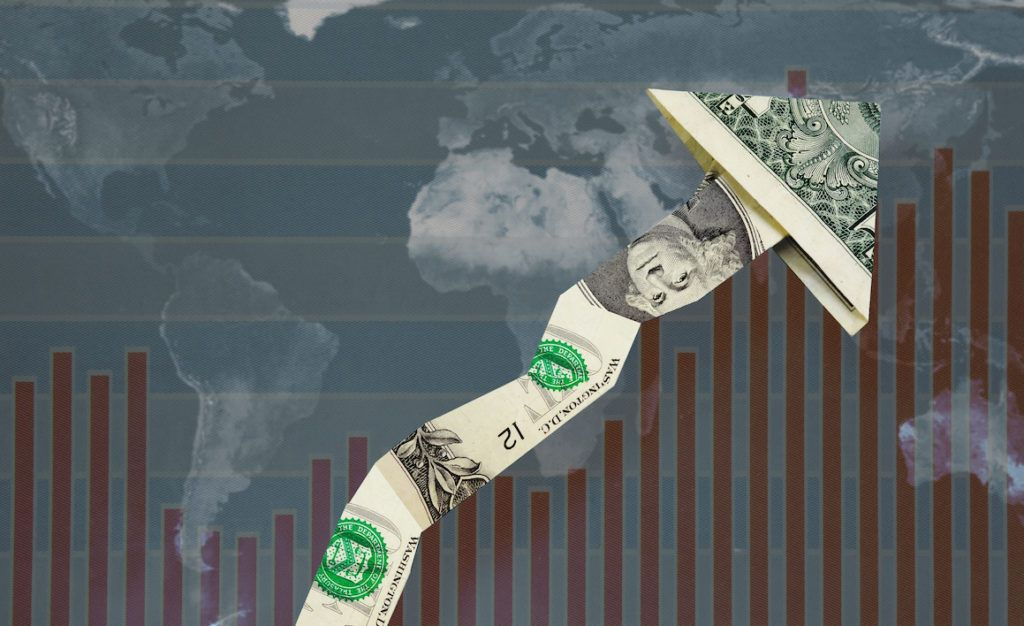The general formula for the price index is the following:

#### PI1,2 = f(P1,P2,X)

Where:

• PI1,2: Some PI that measures the change in price from period 1 to period 2
• P1: Price of goods in period 1
• P2: Price of goods in period 2
• X: Weights (the weights are used in conjunction with the prices)
• f: General function

### Laspeyres Price Index

Ernst Louis Etienne Laspeyres (1834-1913) was a German economist and statistician. Laspeyres’s main contribution to economics and statistics was his work on index numbers and calculating inflation. The formula for Laspeyres Price Index is as follows: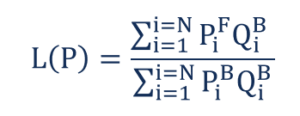Where:

• PiB: The price of good i in the Base period
• PiF: The price of good i in the Final period
• QiB: The quantity consumed of good i in the Base period
• QiF: The quantity consumed of good i in the Final period

The Laspeyres PI weighs prices (both Base period prices and Final period prices) with Base period quantities. Consider an economy with N goods and services. The numerator in the Laspeyres price index calculates nominal expenditure required to consume Base period quantity at Final period prices. The denominator calculates nominal GDP in the Base period.

### Paasche Price Index

Hermann Paasche (1851-1925) was a German economist and statistician. Paasche’s main contribution to economics and statistics was his work on wage inflation.

The formula for Paasche Price Index is as follows: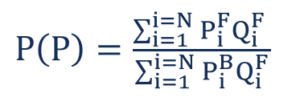Where:

• PiB: The price of good i in the Base period
• PiF: The price of good i in the Final period
• QiB: The quantity consumed of good i in the Base period
• QiF: The quantity consumed of good i in the Final period

Paasche PI weighs prices (both Base period prices and Final period prices) with Final period quantities. Consider an economy with N goods and services. The numerator calculates nominal GDP in the Final period. The denominator in the price index calculates nominal expenditure required to consume Final period quantities at Base period prices.

### Marshall-Edgeworth Index

Alfred Marshall (1842-1924) was an English economist who is widely considered to be the father of modern neoclassical economics. Marshall’s book, Principles of Economics (1890), is one of the most influential textbooks in the history of economic thought. Francis Ysidro Edgeworth (1845-1926) was an Anglo-Irish economist and philosopher. Edgeworth was one of the earliest proponents of using statistics to analyze economic questions.

The formula for the Marshall-Edgeworth Price Index is as follows: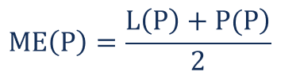Where:

• L(P): The Laspeyres Price Index
• P(P): The Paasche Price Index

The Marshall-Edgeworth Price Index is the arithmetic mean (simple average) of the Laspeyres Price Index and the Paasche Price Index.

### Fisher Price Index

Irving Fisher (1867-1947) was an American economist and statistician. Fisher was one of the earliest neoclassical economists in the US and is known as the first ever econometrician (application of linear regression to economic theory). The American worked on many areas of economics, including trade, monetary theory, and inflation measurement.

The formula for the Fisher Price Index is as follows: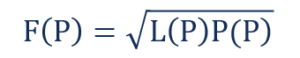The Fisher Price Index is the geometric mean of the Laspeyres Price Index and the Paasche Price Index.

### Illustrative Example

CommodityBase Price (PB)Base Quantity (QB)Final Price (PF)Final Quantity (QF)
110100014800
2122000161500
33050030450
4355000308000
54040045150
6100350150200
725001002000200
8551000502000
910000251050025
10254000403000

Price IndexNumerical Value
Laspeyres Price Index1.025806452
Paasche Price Index0.943342444
Marshall-Edgeworth Price Index0.984574448
Fisher Price Index0.983710712

CFI is the official provider of the Financial Modeling and Valuation Analyst (FMVA)™ certification program, designed to transform anyone into a world-class financial analyst.

To keep learning and developing your knowledge of financial analysis, we highly recommend the additional resources below:

• Phillips Curve
• Price Floors and Ceilings
• Profitability Index
• Technical Analysis – A Beginner’s Guide

### Financial Analyst Training

Get world-class financial training with CFI’s online certified financial analyst training program!

Gain the confidence you need to move up the ladder in a high powered corporate finance career path.

Learn financial modeling and valuation in Excel the easy way, with step-by-step training.